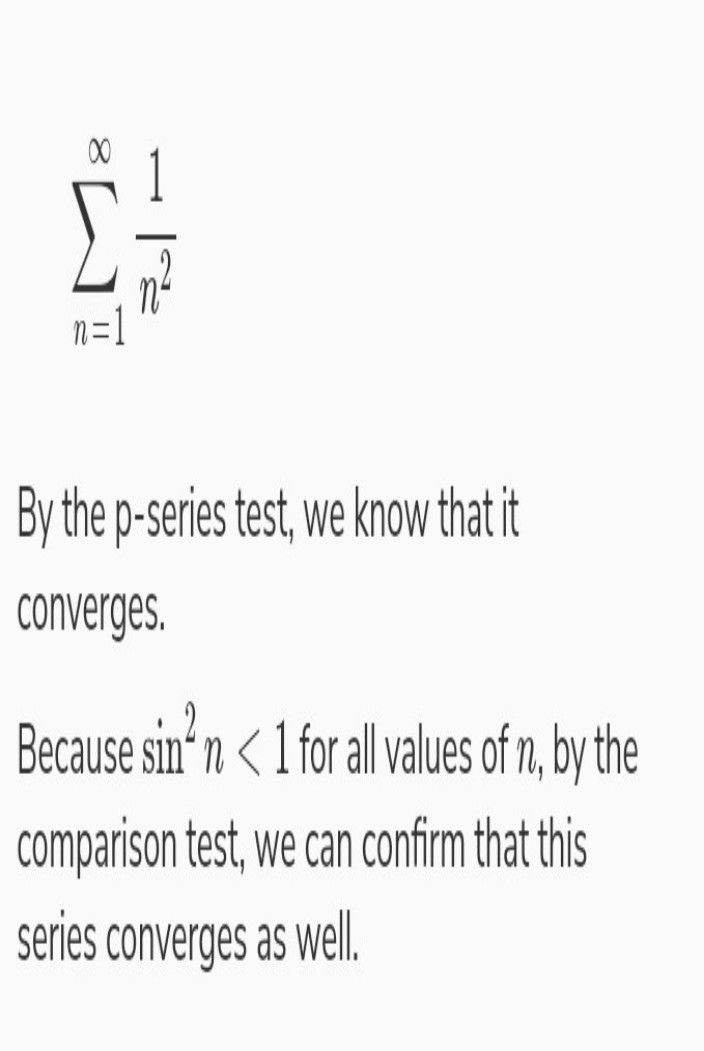Symbol
Problemd) $\right)$ Test the convergence of the series $\sum _{n=1} ^{\infty }\left(\dfrac {n} {2n+1}\right)^{7}$
Algebra
Search count: 112
SolutionQanda teacher - Varun